American Journal of Modern Physics
Volume 4, Issue 6, November 2015, Pages: 296-303

Study of the Zeeman Structure and the Gyromagnetic Ratios of the 2p4f and 3p4f Configurations of the Carbon and Silicon Atoms

Galina Pavlovna Anisimova1, Olga Aleksandrovna Dolmatova1, Anna Petrovna Gorbenko1,
Igor Ratmirovich Krylov1, *, Igor Cheslavovich Mashek1, Martin Luter Tchoffo2,
Galina Aleksandrovna Tsygankova1

1Physics Department, Saint-Petersburg State University, Saint-Petersburg, Russia

2Mesoscopic and Multilayer Structure Laboratory, Department of Physics, Faculty of Science, University of Dschang, Dschang, Cameroon

Email address: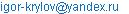(I. R. Krylov)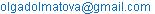(O. A. Dolmatova)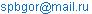(A. P. Gorbenko)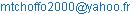(M. L. Tchoffo)

To cite this article:

Galina Pavlovna Anisimova, Olga Aleksandrovna Dolmatova, Anna Petrovna Gorbenko, Igor Ratmirovich Krylov, Igor Cheslavovich Mashek, Martin Luter Tchoffo, Galina Aleksandrovna Tsygankova. Study of the Zeeman Structure and the Gyromagnetic Ratios of the 2p4f and 3p4f Configurations of the Carbon and Silicon Atoms. American Journal of Modern Physics. Vol. 4, No. 6, 2015, pp. 296-303. doi: 10.11648/j.ajmp.20150406.17

Abstract: The present article is a continuation of the authors works devoted to the theoretical study of the fine structure parameters, and other atom characteristics, for which there are no experimental data except for energies of levels of the fine structure. The authors have studied Zeeman structure of the 2p4f and 3p4f configurations and revealed its particular features — crossings and anticrossings of the magnetic sublevels. From splittings of levels in the assured linear range, the authors have calculated gyromagnetic ratios and compared them with their counterparts in the absence of the field. The study of the Zeeman structure is interesting in its own right. Furthermore, through Zeeman splitting in the linear domain of the magnetic field, one can determine the gyromagnetic ratios — one of the most important characteristics of the atoms. Calculation of the Zeeman structure is correct, if in the absence of the field, during the diagonalisation of the energy operator matrix, one obtains the calculated energies, practically coinciding with experimental values (zero energy residuals). To this effect it is necessary to know the numerical values of fine structure parameters. Their exact calculation is possible, if in the energy operator matrix one takes into account not only the electrostatic interaction and the spin-own orbit interaction, where the majority of authors are limited, but also the magnetic interactions, namely: spin-other-orbit, spin-spin, and also the orbit-orbit interactions. Consideration of these interactions is very important for the obtaining null residuals in energy. It is known that, by increasing the role of the magnetic interactions, a deviation from LS-coupling is observed. This is realize in the studied 2p4f C I and 3p4f Si I systems. Authors executed calculations in the jK-coupling approximation taking into account the doublet character of the energy spectra of the considered systems. Later the numerical value of fine structure parameters were introduced in the energy operator matrix; written in the LK and LS-coupling approximations. This was very useful, as gyromagnetic ratios, calculated by intermediate coupling coefficients in different basis, do not always coincide with each other. The comparison of g-factors, determined by different bases in the absence of the field, with the gyromagnetic ratios, calculated by Zeeman splitting was necessary.

Keywords: Fine Structure, Zeeman Splitting, Crossings and Anticrossings of Magnetic Components, Gyromagnetic Ratios, Energy Operator Matrix

1. Introduction

For the higher excited 2p4f C I and 3p4f Si I configurations, there are no experimental data, except the fine structure energy levels [1-3]. Nevertheless, the energies are the best experimental material for the theoretical study of the fine structure parameters and other characteristics of atoms by the semiempirical method. The study of the Zeeman structure is important, in particular, its features — the crossings and the anti-crossings of magnetic sublevels, and also the determination of the gyromagnetic ratios at all levels of the configurations for Zeeman splitting in the linear range of magnetic field. The correct study of Zeeman structure is possible, if in the absence of the magnetic field, at the diagonalization of the energy operator matrix the calculated energies are almost coincide with the corresponding experimental data (zero energy residuals). The high precision calculations are guaranteed by the right mathematical description of the energy levels. To this purpose it is necessary to take in to account in the energy operator matrix, not only the electrostatic interaction and the spin – own orbit interaction, as many authors do, but other interactions, in particular the spin – other orbit, the spin – spin and orbit – orbit interactions. These interactions in the npn`f and the np5n`f configurations were the object of study in our articles [4-8].

2. Energy Spectra of the 2p4f and 3p4f Configurations

We consider in the present work the npnf configurations with the same value of the principal quantum number n of the f-electron, in order to track how the characteristics of the same configurations change with increase of nuclear charge. Earlier the Zeeman structure of similar configurations with n’=5 were considered in the works: 3p5f Si I  and 2p5f C I .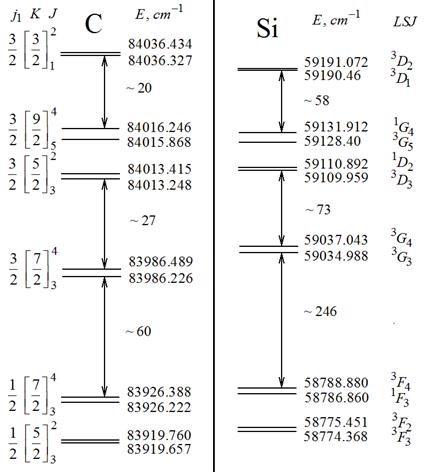Figure 1. Energy spectra of the 2p4f (left) and 3p4f (right) configurations of the carbon and silicon atoms.

The energy spectra of the considered configurations are presented on Fig. 1. The classification of the levels of the carbon atom is given in the approximations of LK-coupling  and jK-coupling , the silicon atom in the approximation of jK-coupling . On the figure the notations of the levels in the approximation of LS-coupling are also indicated. The LS-coupling approximation is the most convenient and compact in the writing. In it is clearly seen, the singlet and the triplet levels. Let’s indicate that, the numerical calculation of the fine structure parameters was effected in jK-coupling approximation with respect to the above cited works [2-3]. Laterthe obtained numerical values of the fine structure parameters were introduced in the energy operator matrices, written in the LS and LK coupling approximations (see below). This gave the possibility to compare the classification of levels in different approximations.

In the case of fitting the LS-coupling, the energy spectrum is constituted of triplets and singlets, and triplet components are close to each other, while the singlet level is significantly far from the triplet. From Fig. 1 it is seen that such a situation is not realized in the considered systems. In both configurations the pairing of levels is clearly seen. In the lower part of the spectrum there are F-levels with j1 = ½ (j1 = l1 + s1). In the LS-coupling the disposition of the levels of the normal triplet must be as follows: 3F2 (the lower level), followed by higher levels 3F3, 3F4; 1F3 is the upper singlet level. In the considered 2p4f and 3p4f configurations, in both pairs, the levels changed places.

On the upper part of the energy spectrum on Fig. 1 there are 4 pairs of G and D-levels with j1=3/2. The G and D-levels are alternated. In general spectrum the 2p4f configurations of carbon and 3p4f of silicon are similar. Let’s notice that, the 2p4f configuration occupies an energy interval of 116 cm-1, while the 3p4f configuration occupies 417 cm-1, that means 3.6 times greater than that of carbon. Both configurations are isolated from other configurations of the same parity (for instance npnp). In these configurations, there are some levels of np4d configuration [11,12], but this configuration has different parity as compared to the considered np4f configuration and does not interact with them.

3. Energy Operator Matrix and the Fine Structure Parameters

The energy operator matrix of npnf configurations (and also np5nf) is obtained in two representations: LSJM (LS-coupling approximation) and independent momentums by the general form formulae from the monograph . In the LSJM- representation it is differentiated by the quantum number J (the total atom electron moment), in the independent moment representation ( author’s terms) it is differentiated by the magnetic quantum number M. Representation of independent momentums is convenient, due to the fact that in it the states of two-electron atom (two electrons in the outer shells) depend only on individual quantum numbers of single electrons. Furthermore, the energy operator matrix of np5n`f hole configurations (realized in rare gasses starting from neon) can be obtained only in representation of independent momentums, by changing in the corresponding wave functions the sign of the orbital and spin projections of p-electron. Thus, in the p5f hole configuration one also considers two particles, f-electron and p-hole (almost full shell).

All angular momentums (l1, l2, s1, s2, L, S, J) are irreducible tensor operators of first rank . The Wigner–Ekkart theorem , which states that the matrix element is the product of phase factor, of reduced matrix element and of the Wigner’s 3j-symbol, can be applied to them. As all the formulae  in the independent momentums are written through the orbital and spin unitary operators, then the reduced matrix element is equal to unity. That is why all the calculation is reduced to calculation of the 3j-symbol, which is easier, than the calculation of Wigner’s 9j-symbol in the LSJM representation (see details in articles [4-8,16]).

The matrix elements, calculated with wave functions of independent momentums representation, were transformed later to the LSJM-representation with the help of the matrices of the coefficients of wave function transformation of one representation through the wave functions of the other representation . Then it's were compared with the results of independent calculations in the LSJM-representation . Calculations results for the electron npn`f configuration must totally coincide, which was attained in our investigation.

Table 1. Energy Operator Matrix of the npn`f configurations in LSJM-representation.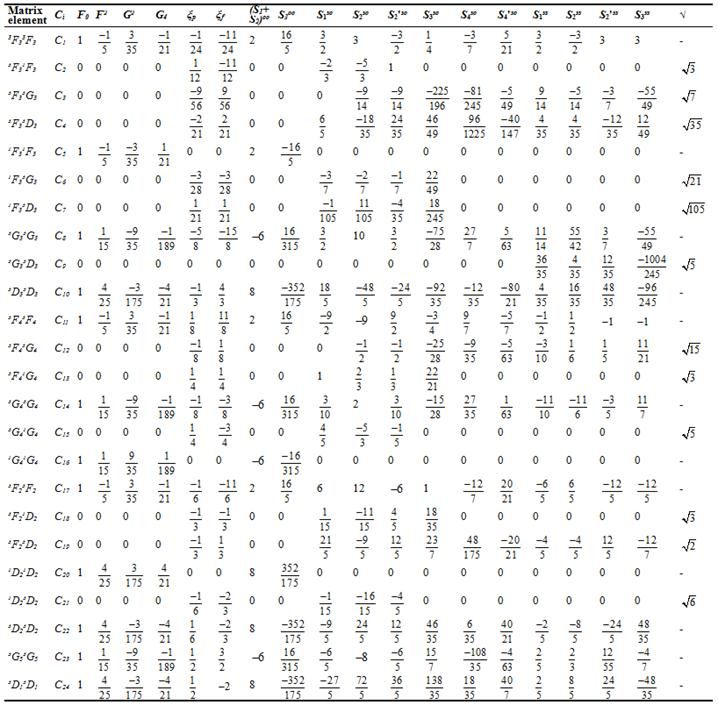The energy operator matrix of considered configurations is presented in Table 1 in the form of coefficients at radial integrals (called later fine structure parameters). Every matrix element is a linear function of fine structure parameters with the corresponding coefficients. The square root is the common factor for all rows of coefficients in nondiagonal matrix elements in Table 1.

Let’s describe the radial integrals. In Table 1, F0, F2 and G2, G4 are direct and exchange by Slater’s integrals (electrostatic interaction); ξp and ξf are radial integrals of the spin - own orbit interaction; S1, S2, S2` are direct radial integrals of Marvin Mk-1 spin interactions , S3 is the Marvin exchange radial integral Nk-1 ; S4 and S4` are exchange radial integrals Kk, related to Marvin integrals. Affiliation Marvin integrals to concrete interactions is denoted by the upper indices: so — for spin – other orbit interaction, ss — for spin – spin interaction, oo — for orbit – orbit interaction. Thus the energy operator matrix is constituted of 18 radial integrals, which are the fine structure unknown parameters in the semiempirical calculation. They are determined numerically from the solution of the system of nonlinear equations on the unitary transformation of nondiagonal Hermitian matrix to the diagonal form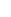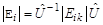(1)

whereis a diagonal matrix (in our case it’s the experimental value of the energy on Fig. 1);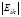is a nondiagonal matrix from Table 1 (symmetric matrix elements are not written);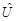is a unitary matrix of transformation coefficients (below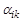, where i is the row number, k – the column number). In other wordsare coefficients of expansion of wave functions of intermediate (real) couplings by wave functions of any vector type of coupling.

In any vector coupling approximation (LS, LK, jK, jj) the energy operator matrix is differentiated by the quantum number J. In the systems of 12 levels (in our case npnf) we have one matrix of rank 4 (J = 3), two matrices of rank 3 (J = 2, 4) and two matrices of first rank (J = 5 and J = 1).

The number of equations in (1) is determined by the rank of the matrix n, and equal to n2. In the analytic form the equations are obtained if equation (1) multiply the right side by matrix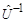, then multiply the two matrices in both sides of (1) and equate corresponding elements. The system of equations obtained in this manner is sufficiently simple, as it is basically constituted of quadratic equations. The number of equations in (1) are exactly equal to the number of coupling coefficients, which are unknown values as the fine structure parameters. That is why the system of quadratic equations in (1) has been supplemented by equations of normalization and orthogonality of coupling coefficients for the correspondence of the number of equations and the number of unknowns. Finally by the Newton's iteration method the system of 52 nonlinear equations for 52 unknown values (18 fine structure parameters and 34 coupling coefficients) were solved. The known values are the energy levels of the fine structure.

The Newton’s iteration method requires the null approximation. The first null approximations are determined from the solution of the system of 5 linear equations by Slater’s diagonal sum rule (the trace of matrices) for 5 fundamental parameters: F0, F2, G2, G4, ξp. Later the numerical values of these parameters were put into the matrix elements from Table 1 (the other parameters were supposed to be equal to zero) and matrices of the above cited rank were diagonalized. As a result the calculated energies (eigen values) and coupling coefficients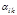(eigen vectors) were determined. Knowing the coupling coefficients and the numerical values of elements of diagonal matrix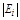(experimental energies), by formula (1) one can determine the numerical values of elements of nondiagonal matrix(in Table 1 designated by Ci). Thus the system of 24 linear equations for 18 unknown fine structure parameters was obtained. It was solved by least square method (LSM). As a result, the zeroth approximations for all parameters were determined. The cycle of calculations was repeated until the discrepancy for energies became small, such that the Newton’s iteration method started to converge. Let’s pay attention on the fact, that in every step of the calculations, the numerical diagonalization of energy operator matrices were executed, that means all the calculations were done in the intermediate coupling.

As mentioned above, the calculation of fine structure parameters were executed in the jK-coupling approximation with respect to the classification levels [2, 3]. For this the purpose so many time verified by two representations of energy operator matrix from Table 1, written in the LS-coupling approximation, was transferred into the jK-coupling approximation (and also into the LK-coupling) with the help of the corresponding coefficients of transformation of wave functions from one representation to another. The corresponding formulae were borrowed from  and were published in our article  (formulae (2), (3)). For the npnf configuration the coefficients of transformation LS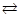jK and LSLK are presented in Table 2 together with the corresponding vector g-factors. The latter were calculated with respect to formula: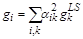(2)

whereare coefficients from Table 2. The energy operator matrix in the jK-coupling approximation is not shown. It's easy to obtain from data of Table 1 and 2.

The fine structure parameters, determined with respect to the described technique, are presented in Table 3 in comparison with a few data from the work  for carbon atom. The table shows that the spin-orbit splitting constant ξpof silicon atom is 4 times greater than the similar value for carbon atom. All the rest of parameters of siliconalso increase as compare to carbon. This explains the much large energy spectrum range of the 3p4f configuration of silicon as compared to the 2p4f configuration of carbon atom (see Fig. 1).

Table 2. Transformation coefficients of the wave function of the one type coupling through the wave functions of the other type coupling and the corresponding gyromagnetic rations for nsn'f configurations.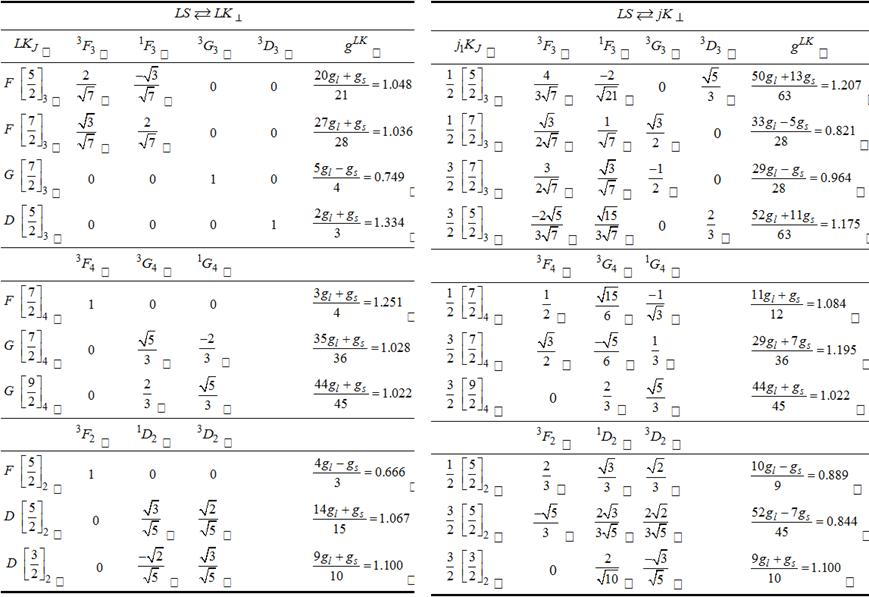LS-coupling gyromagnetic ratios: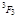= 1.08353,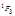= 1.0,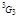= 0.74942,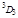= 1.33411;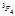= 1.25058,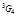= 1.05012,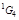= 1.0;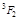= 0.66589,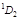= 1.0,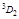= 1.16705.

Table 3. The fine-structure parameters of the 2p4f and 3p4f configurations.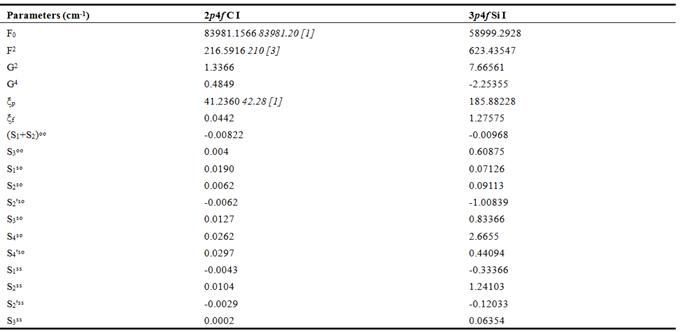4. Zeeman Splitting

4.1. The Particularities of Zeeman Structure

The magnetic field completely removes the degeneracy of levels with respect to quantum number M. The energy operator matrix is differentiated by M: M = ± 5 is a matrix of first rank, М = ± 4 is a matrix of fourth rank, М = ± 3 is a matrix of eighth rank, М = ± 2 is a matrix of eleventh rank, М = ± 1 and М = 0 are matrices of twelve rank.

In order to determine the particularities of Zeeman splitting (the crossings and the anticrossings of Zeeman components) the numerical diagonalisation of all indicated submatrices was performed. The most convenient and compact in writing is the energy operator matrix in the LSJM representation (LS-coupling approximation) — see Table 1 and the fine structure parameters in Table 3. It’s necessary to add to it the elements of the energy operator matrix of interaction of the atom with the magnetic field. The corresponding operator, and also diagonal and non diagonal matrix elements, was published in our article  (see formulae (13) – (15) and the comments on them). The results of calculations of matrix elements with respect to the cited formulae for the npn’f configuration were published in our article  (Table 2). There is one error. In the column with M = 0 on Table 2  one must read: 3G53G4 = (2/3) (glgs) μ0H without 5 in parenthesis. All non diagonal matrix elements of similar matrices have the factor (glgs).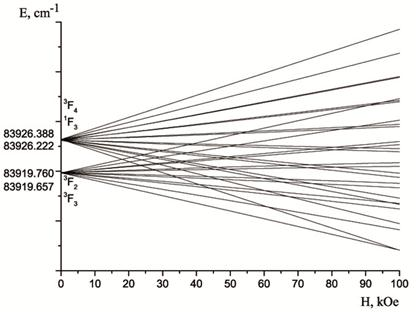Figure 2. Energy spectra of the 2p4f (upper) and 3p4f (lower) configurations of the carbon and silicon atoms.

The dependences of Zeeman energy sublevels of the considered configurations: 2p4f and 3p4f were studied in the (1-100) kOe range of the magnetic field and were built graphically firstly with a big increment of the field through 10 Oe. The general view of Zeeman splitting of the considered systems is shown on Fig. 2. Later, in the range of crossed components with ΔM = ±1, ±2 the position of the minimum were fixed at condition EiEk = 0.0001 cm-1 (Ei and Ek are the energies of crossed components). The results of these studies are presented in Table 4 (the first 30 crossings). It is seen that in carbon the crossings start earlier as compared to silicon, the energy range of 2p4f configuration is much less then that of 3p4f Si I configuration. Furthermore, the sequence of crossings is changing in both configurations. The number of crossings in the (1-100) kOe range for 2p4f C I configuration is 104, and the same number of crossings for 3p4f Si I configuration is 51.

Unfortunately, there are no similar experimental data. The results of Table 4 are prognosis for future experiments.

Apart from the crossing of magnetic components in the studied configurations, one observes the anticrossing sublevels with ΔM = 0. Results for the 1F3 and 3F2 levels are presented on Firg.3 and in Table 5. It is known that, the Zeeman components of different terms are anticrossing. In the LS-coupling approximation 3F2 and 1F3 are different terms (triplet and singlet). In the jK-coupling approximation the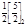and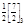levels are also different terms. They have different intermediate momentums K (see Fig. 1). There are anticrossings of other pairs of levels, but they are in very great fields. In this article we don’t consider them.

Table 4. The fields of the crossings for the Zeeman sublevels with ΔM = ± 1, ± 2.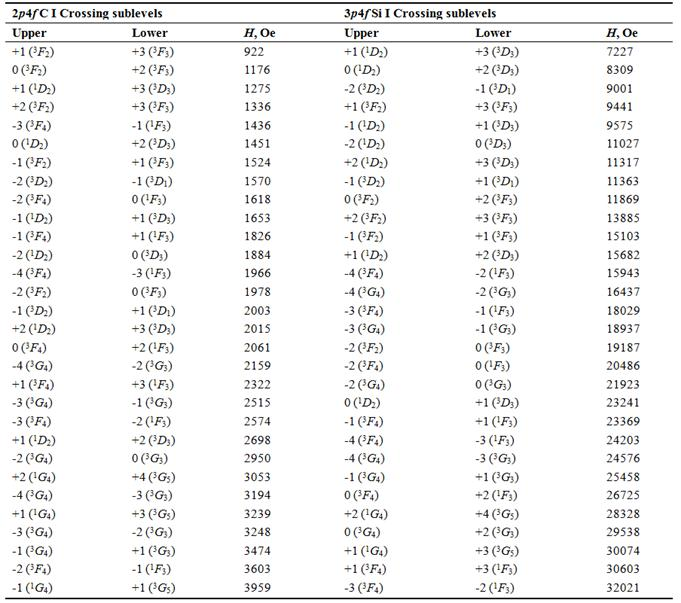4.2. The Gyromagnetic Ratios

Let’s explain, why is it necessary to determine the gyromagnetic ratios with respect to Zeeman splitting. As it was said earlier, the calculation of fine structure parameters was executed in the jK-coupling approximation. Later the numerical values of fine structure parameters from Table 3 were put in the energy operator matrix in the LK and LS-coupling approximations and their numerical diagonalisation were performed in the absence of the field (H=0). As a result, the calculated energies and the intermediate coupling coefficients αik were obtained.

Table 5. Minimal energy intervals and corresponding magnitudes of magnetic fields in waists of anticrossings.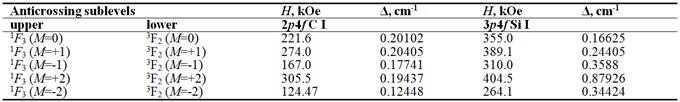With these coefficients by formula (2) the gyromagnetic ratios for 10 levels of configuration with respect to all three bases: LS, LK, jK, were calculated. It seems that only for two levels 1G4 and 3D2, the g- factor is the same in all three considered bases and coincide with their vector counterparts in the jK-coupling.

This tells how close the indicated levels are to the jK-coupling, in the approximation in which the calculation of fine structure parameters are performed. For the rest of the 8 levels the g-factors are practically the same for two bases; LS and LK. For the jK basis, they are different (see Table 7). In order to determine which g-factors when H=0 are authentic, we calculated them with respect to the Zeeman splitting. Such calculation is true only in the linear part of the magnetic field. The condition of linearity is: energy intervals between magnetic components with M = +1, M = 0 and M = -1, M = 0 must be the same. The magnetic field H = 300 Oe was chosen. Table 6 convincible demonstrates that the indicated condition is fulfilled. In order to determine the intermediate coupling coefficients and respectively the gyromagnetic ratios, it is sufficient to effect the numerical diagonalisation of the 12 rank matrix for one value of M, for instance М = +1 (or M = -1, M = 0).

Table 6. Demonstration of the linearity of Zeeman sublevels when H= 300 Oe.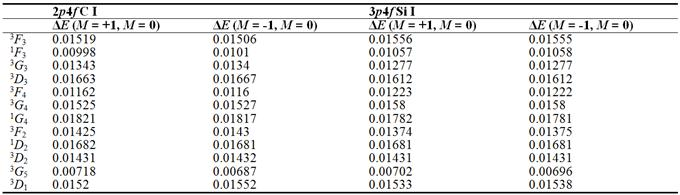Table 7. The gyromagnetic ratios in three bases LS, LK, jK in the absence of magnetic field and when H = 300 Oe.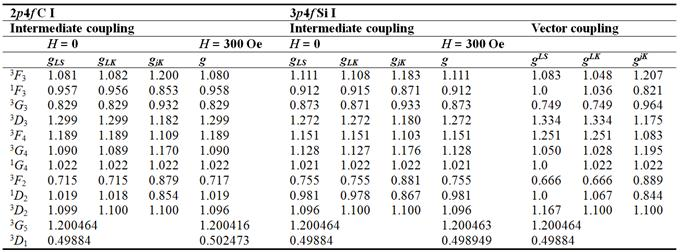The results of the calculation are presented in the summarized form on Table 7, together with g-factors when H = 0 and their vector counterparts for the appreciation of the character of coupling in the studied systems. It is observed that, when H = 300 Oe the g-factors are practically the same with g-factors of LS and LK bases. Unconditionally the 1G4 and 3D3 levels belong to jK-coupling (coincidence of g-factors with respect to all three basis).

In general the majority of the levels of 2p4f C I configurations are near to LK-coupling. That is why the authors of the work  gave the classification of the levels in this approximation LK-coupling. The 3p5f Si I configuration has 5 levels (1G4, 3D2, 3G3, 3F4, 3G4) near to jK-coupling; the 3F3, 3D3, 1D2 levels are near to LK-coupling; the 1F3 and 3F2 levels occupy an intermediate position between LK and jK- coupling types. That means the considered system as a whole is near to jK-coupling. Consequently, the classification of levels in this approximation in work  is justified.

Let’s consider separately the 3G5 and 3D1 levels with the unique value of quantum number J in the considered systems. These levels are supposed to be independent of the type of coupling, and their g-factors are the same in all types of coupling, including the intermediate coupling. In fact it is not like that. The 3D1 С I level shows a shift from LS-coupling already in third sign, the 3G5 level is shifted insignificantly from LS-coupling (in fifth sign). For the atom of silicon the 3D1 level is shifted from LS-coupling in fourth sign; the g-factor of the 3G5 Si I levels are the same for (H = 0) and for (H = 300 Oe).

Thus, the calculations of gyromagnetic ratios with respect to Zeeman splitting confirm our conclusion, that if at least two g-factors out of three with respect to different basis coincide, then they can be considered authentic. The coincidence of g-factors for three basis is an ideal variant of the closeness of the level to this approximation, in which the calculations of the fine structure parameters were done.

References

1. L. Johansson, Spectrum and term system of the neutral carbon atom, Arkiv för Physik 31 (15), 201 (1966).
2. Edward S.Chang, and Murray Geller, Improved Experimental Energy Levels of Carbon I from Solar Infrared Spectra, Phys. Scr. 58, 330 (1998).
3. L. J. Radziemski, K. L. Andrew, V. Kaufman, and U. Litzen, Vacuum Ultraviolet Wavelength Standards and Improved Energy Levels in the Fist Spectrum of Silicon, J. Opt. Soc. Am. 57 (3), 336 (1967).
4. G.P. Anisimova, and E.L. Kapel`kina, Spin-Other-Orbit Interaction in Two-Electron Configurations with p and f Electrons: Direct Terms, Optics and Spectroscopy 84 (3), 313 (1998).
5. G.P. Anisimova, and E.L. Kapel`kina, Spin-Other-Orbit Interaction in Two-Electron Configurations with p and f Electrons: Excharge Terms, Optics and Spectroscopy 84 (4), 476 (1998).
6. G.P. Anisimova, and E.L. Kapel`kina, Two-Electron Matrices of the Total Energy Operator of the Spin-Orbit Magnetic Interaction for pf, p5f, fp, and f13p Configurations, Optics and Spectroscopy 84 (6), 799 (1998).
7. G.P. Anisimova, and E.L. Kapel`kina, Account for the Spin-Spin Interaction in the Energy Matrices of Two-Electron Configurations with p and f Electrons, Optics and Spectroscopy 87 (1), 9 (1999).
8. G.P. Anisimova, and E.L. Kapel`kina, Orbit-Orbit Interaction in Two-Electron Matrices of the Energy Operator for pf Configurations, Optics and Spectroscopy 87 (6), 805 (1999).
9. G.P. Anisimova, O.A. Dolmatova, M. Choffo, Determination of Gyromagnetic Ratios from the Zeeman Splitting of Levels of the 3p5f Configuration of the Silicon Atom,Optics and Spectroscopy114 (2), 177 (2013).
10. G.P. Anisimova, I.Ch. Mashek, O.A. Dolmatova, A.P. Gorbenko, R.I. Semenov, and M.L. Tchoffo,Features of the Zeeman splitting and g-factors of 2p5f configuration levels of carbon atom,American Journal of Modern Physics 3 (6), 218 (2014).
11. NIST Atomic Spectra Database Levels Data. C I 282 Levels Found. 2009.
12. NIST Atomic Spectra Database Levels Data. Si I 542 Levels Found. 2008.
13. A.P. Yutsis, and A.Yu. Savukinas, Mathematical Foundations of the Theory of Atom (Vil`nyus, 1973) [in Russian].
14. D. A. Varshalovich, A. N. Moskalev, and V. K. Khersonskii, Quantum Theory of the Angular Moment, Leningrad, 1975 [in Russian].
15. I.I. Sobelman, Introduction to the Theory of Atomic Spectras (Moscow, 1963) [in Russian].
16. G. P. Anisimova, O. A. Dolmatova, and I. S. Rusnak, Spin–Other Orbit Interaction in Highly Exited Configurations with p and g Electrons in Outer Shells, Optics and Spectroscopy 107 (4), 545 (2009).
17. G.P. Anisimova, I. Ch. Mashek, O. A. Dolmatova, A.P. Gorbenko, R. I. Semenov, M. Tchoffo, G.A. Tsygankova,The Fine-Structure Parameters and Zeeman Splitting of Levels of the Configurations 1sni (n = 7 − 10) of the Helium Atom, American Journal of Modern Physics 3 (4), 143 (2014).
18. J. B. Green, and J. F. Eichelberger, The Pashen-Back Effect. Theory of the Effect for Intermediate Coupling, Phys. Rev. 56 (1), 51 (1939).
19. V. Kaufman, and J. Sugar, Wavelengths and Energy Level Classifications of Scandium Spectra for All stages of Ionization, J. Phys. Chem. Ref. Data 17 (4), 1679 (1988).

 Contents 1. 2. 3. 4. 4.1. 4.2.
Article ToolsAbstractPDF(817K)
Follow on us
PUBLICATION SERVICE
JOIN US
RESOURCES
SPECIAL SERVICES
ADDRESS
Science Publishing Group
548 FASHION AVENUE
NEW YORK, NY 10018
U.S.A.
Tel: (001)347-688-8931